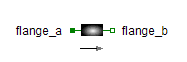Multiphase Capacitor - MapleSim Help

Multiphase Capacitor

Ideal linear electrical capacitorsDescription The Multiphase Capacitor (or Capacitor) component contains $m$ single-phase capacitor models, each connected between corresponding phases of ${\mathrm{plug}}_{p}$ and ${\mathrm{plug}}_{n}$.Connections

 Name Description Modelica ID ${\mathrm{plug}}_{p}$ Positive $m$-phase plug plug_p ${\mathrm{plug}}_{n}$ Negative $m$-phase plug plug_nParameters

 Name Default Units Description Modelica ID $m$ $3$ $1$ Number of phases m $C$ $\left[1,1,1\right]$ $F$ Capacitance vector CModelica Standard Library The component described in this topic is from the Modelica Standard Library. To view the original documentation, which includes author and copyright information, click here.